# Practice Questions: Electricity Notes | Study Science Class 10 - Class 10

## Class 10: Practice Questions: Electricity Notes | Study Science Class 10 - Class 10

The document Practice Questions: Electricity Notes | Study Science Class 10 - Class 10 is a part of the Class 10 Course Science Class 10.
All you need of Class 10 at this link: Class 10

1. What is the difference between a conductor and an insulator? Give one example of each.
2. The current in a wire is one ampere. Explain this statement in terms of the charge flowing through the wire.
3.  When do you say that the resistance of a wire is 1W?
4. Draw a circuit diagram for a circuit in which two resistors A and B are joined in series with a battery, and a voltmeter is connected to measure the potential difference across the resistor A.
5. When are resistors said to be connected in series?
6. When are resistors said to be connected in parallel?
7. Why is tungsten suitable for making the filament of a bulb?
8. Why is tungsten not used as a fuse wire?
9. Alloys are preferred over metals for making the heating element of heaters. Why?
10. Silver is a better conductor of electricity than copper. Why then do we use copper wire for conducting electricity?
11. State Ohm's law. How can it be verified?
12. When the terminals of a cell are connected to the ends of an iron rod, electric current flows through the rod. When the terminals are connected to the ends of a wooden rod, no current flows. Explain why, when the wooden rod also has a large number of electrons.
13. Define electric current and state its unit. How can Ohm's law be used to define ohm?
14. Deduce the expression for the equivalent resistance of the parallel combination of two resistances R1 and R2.
15. Deduce the expression for the equivalent resistance of the two resistances R1 and R2 connected in series.
16. Derive an expression for the heat produced in time t in a wire of resistance R, which is carrying a current

Numericals

1. How much work will be done in bringing a charge of 5.0 milicoulombs from infinity to a point P at which the potential is 12V ?

2. A particle with a charge of 1.5 coulombs is taken from a point A at a potential of 50V to another point B at a potential of 120V. Calculate the work done.

3. How many electrons are required to get 1C of negative charge ?

4. Calculate the current in a wire if 900C of charge passes through it in 10 minutes.

5. How much current will flow through a resistor of resistance 12W if a battery of 18V is connected across it?

6. Calculate the resistance of a copper wire of length 1m and area of cross section 2 mm2. Resistivity of copper is 1.7 × 10 -8 W m.
7. A copper wire has a resistance of 0.5 W. Another copper wire of the same mass as the first one is double in length of the first. Find the resistance of the second wire.

8. In an experiment to verify Ohm' law, the current through a resistor and the potential difference across it are measured. From the values given below, plot a graph of i versus V. Show that the data confirms Ohm's law, and find the resistance of the resistor.

Current (A) 0.1, 0.2, 0.3, 0.4

Potential difference (V) 1.2, 2.4, 3.6, 4.8

9. When a potential difference of 20 V is applied across a resistor, it draws a current of 3A. If 30V is applied across the same resistor, what will be the current.

10. How will the resistance of a wire change if its diameter (d) is doubled, its length remaining the same ?

11. Calculate the potential difference across each resistor in the circuit shown in figure.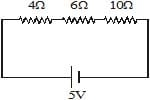12. Three identical bulbs are connected in parallel with a battery. The current drawn from the battery is 6A. If one of the bulb gets fused, what will be the total current drawn from the battery ?

13. A uniform wire of resistance R is cut into three equal pieces, and these pieces are joined in parallel. What is the resistance of the combination ?

14. Consider the circuit shown in figure. The voltmeter on the left reads 10V and that on the right reads 8V. Find (a) The current through the resistance R, (b) the value of R, and (c) the potential difference across the battery.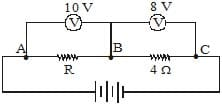15. Three resistors of reistances 10W, 20W and 30 are connected in parallel with a 6V cell. Find (a) The current through each resistor, (b) the current supplied by the cell, and (c) the equivalent resistance of the circuit.

16. Consider the circuit shown in fig. Calculate the current through the 3W resistor.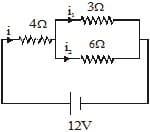17. When two resistors are joined in series, the equivalent resistance is 90W. When the same resistors are joined in parallel, the equivalent resistance is 20W. Calculate the resistance of the two resistors.

18. (a) How will you join three resistors of resistance 4W, 6W and 12W to get an equivalent resistance of 8W.

(b) What would be the highest and the lowest equivalent resistances possible by joining these resistors.
19. How many bulbs of resistance 6 ohms should be joined in parallel to draw a current of 2 amperes from a battery of 3 volts.

20. A current of 4A passes through a resistance of 100W for 15 minutes. Calculate the heat produced in calories.

21. A 12V battery is connected to a bulb. The battery sends a current of 2.5A through it. Calculate

(a) the power delivered to the bulb, and (b) the energy transferred to the bulb in 5 minutes.

22. A current is passed through a resistor for some time. It produces 400 cal of heat in this period. If the current is doubled, how much heat will be produced for the same duration.

23. Calculate the wattage of an electric heater which draws 5A current when connected to a 220V power supply.

24. A bulb draws 24W when connected to a 12V supply. Find the power if it is connected to a 6V supply.
(Neglect resistance change due to unequal heating in the two cases.)

25. Two identical resistance R are connected in series with a battery of potential difference V for time t. The resistor are later connected in parallel and the same battery is connected across the combination for time t. Compare the heat produced in the two cases.

26. A bulb is rated 40W, 220V. Find the current drawn by it when connected to a 220V supply.

27. A bulb is rated 60W, 240V. Calculate its resistance when it is on. If the voltage drops to 192V, what will be the power consumed and the current drawn ?

28. A room has two tube lights, a fan and a TV. Each tube light draws 40W, the fan draws 80W, and the TV draws 60W. On the average, the tube lights are kept on for five hours, the fan for twelve hours and the TV for eight hours every day. The rate for electrical energy is Rs. 3.10 per kWh. Calculate the cost of electricity used in this room in a 30 day month.

29. When a particle of charge 10mC is brought from infinity to a point P, 2.0 mJ of work is done by the external forces. What is the potential at P ?

30. Calculate the work done in taking a charge of 0.02C from A to B if the potential at A is 20V, and that at B is 30 V.

31. How much charge flows through a wire in 10 minutes if the current through it is 2.5A ?

32. A 2V cell is connected to a 1W resistor. How many electrons come out of the negative terminal of the cell in 2 minutes?

33. The amounts of charge passing through a cell in 4 seconds is 12C. What is the current supplied by the cell?

34. A 6V battery is connected across a 5W resistor. Calculate the current passing through the resistor.

35. When a 24V battery is connected to a resistor, the current in it is 0.4A. What is the resistance of the resistor? What would be the current through it when it is connected to a battery of 6V ?

36. In an experiment, the current flowing through a resistor and the potential difference across it are measured. The values are given below. Show that these values confirm Ohm's law, and find the resistance of the resistor.

i (ampere) 1.0 1.5 2.0 2.5 3.0

V(volt) 4.0 6.0 8.0 10.0 12

37. The resistivity of copper is 1.7 × 10_8 Wm.

(a) What length of copper wire of diameter 0.1 mm will have a resistance of 34 W.

(b) Another copper wire of same length but of half the diameter as the first is taken. What is the ratio of its resistance to that of the first wire ?

38. Three resistors, each of resistance 12W, are connected in parallel. What is the equivalent resistance ?

39. A uniform wire of resistance R is cut into two equal pieces, and these pieces are joined in parallel. What is the resistance of the combination?

40. You have three resistors of 9 ohms each. By combining them what can be (a) the highest resistance, and (b) the lowest resistance? (c) How can you combine them to get a resistance of 12W?

41. How will you join the resistors of resistance 3W, 6W and 8W to get an equivalent resistance of 10W ?

42. Find the current through the circuit shown in fig. Also find the potential difference across the 20W resistor.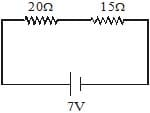43. Find (a) the equivalent resistance, (b) the current passing through the cell, and (c) the current passing through the 30W resistor in the circuit shown in fig.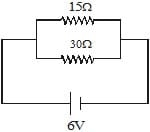44. Find the current supplied by the cell in the circuit shown in fig.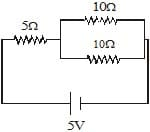45. In the circuit shown below, calculate the total resistance of the circuit and the current flowing through it.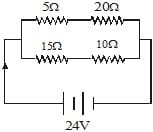46. Fig shows a part of an electric circuit. The reading of ammeter is 3.0A. Find the currents through the 10W and 20W resistors.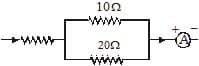47. A 12V battery connected to a bulb drives a current of 2.0A through it. Find the energy supplied by the the battery in 10 minutes.

48. A current of 1.5A flows through a wire of 8W. Find the amount of heat produced in 10 seconds.

49. A current of 2A produces 200J of heat in a wire in a given period of time. If the current is increased to 4A how much heat will be produced in the same time.

50. A bulb is rated 5.0V, 100mA. Calculate its rated power and resistance.

51. Calculate the resistance of a bulb rated 40W, 230V when in ON condition.

52. Calculate the current passing through a bulb rated 60W, 240V when it is connected to a 240V power supply.

53. Two resistors of resistances 10W and 20W are joined in series. A potential difference of 12V is applied across the combination. Find the power consumed by each resistor.

54. Two resistors of resistances 10W and 20W are joined in parallel. A potential difference of 12V is applied across the combination. Find the power consumed by each resistor.

55. Calculate the energy consumed in kilowatt hours by a 60W fan in 2 hours.

56. A heater draws 1100W at 220V.

(a) Find the resistance of the heater when in ON condition.

(b) Calculate the kilowatt hours consumed in a week if the heater is used daily for four hours at the rated voltage.

57. A bulb used in a car is rated 12V, 48W. Find the energy consumed in one minute when the bulb is connected to (a) a 12V battery and (b) a 6V battery.

58. 6 × 107 electrons cross through an area per minute. What is the electric current ?

59.  A 4V battery is connected to a lamp of resistance 4 W. Calculate the current through the lamp.

60. Calculate (a) the equivalent resistance, (b) the electric current, and (c) the potential difference across each resistor in the circuit shown in Figure.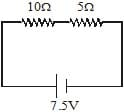61. Two resistances of 3W and 6W are connected in parallel. Calculate their equivalent resistance.

62. A 1W Resistor is connected in parallel to a 10W resistor. Calculate the equivalent resistance.

63. Two resistors of resistances 10W and 20W are connected in parallel. A battery supplies 6A of current to the combination, as shown in Fig. Calculate the current in each resistor.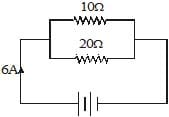64. A 3V battery is connected across a 5W resistance. Calculate the heat produced in 5 seconds.

65. An electric kettle is rated 500W, 220V. It is used to heat water for 30 seconds. Assuming the voltage to be 220V, calculate the heat produced.

1. 0.06J  2. 105J  3. 6.25 × 1018   4. 1.5A  5. 1.5A

6. 8.5 × 10_3W  7. 2.4W   8. 12W   9. 4.5A

11. 1V, 1.5V, 2.5V    12. 4A   13.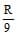14. 2A,5W,18V   15. 5.5W

16. 1.33A   17. 30W   19. 4   20. 3.4 × 105 ca

21. 30W, 9000J    22. 1600 cal    23. 1100 W   24. 6W

26.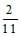A   27. 38.4W, 0.2A   28. Rs.171.12    29. 200V   30. 0.2J

31. 1500C    32. 1.5 × 1021 electrons    33. 3A    34. 1.2A    35. 60W, 0.1A

36. 4W    37. (a) 15.71m, (b) 4:1 (4 times)   38. 4W    39.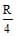40. (a) 27W (b) 3W (c) one resistor connected in series to a combination of two resistors in parallel

41. 8W resistor connected in series to a parallel combination of 6W and 3W

42. 0.2A, 4V    43. (a) 10W (b) 0.6A (c) 0.2A    44. 0.5A    45. 12.5W, 1.92 A

46. 2A, 1A    47. 14,400J    48. 180J or 43 cal    49. 800J   50. 0.5W, 50W

51. 1322.5W    52. 0.25A    53. 1.6W, 3.2W    54. 14.4W, 7.2W   55. 0.12 kWh

56. (a) 44W (b) 30.8 kWh    57. (a) 2880J (b) 720J

58. 1.6 mA.    59. 1A    60. (a) 15 W. (b) 0.5A (c) 5V , 2.5V

61.  2W    62. 0.99W    63. 4A, 2A    64. 9J    65. 15,000 J.

Previous Year Board (CBSE) Questions
Very Short Answer Questions (Carrying 1 Mark each)

1. Define resistivity of a material.  

2. A cylinder of a material is 10 cm long and has a cross-section of 2 cm2. If its resistance along the length be 20W, what will be its resistivity in number and units.  

3. Why is tungsten metal selected for making filaments of incandescent lamps?  

4.  A resistance of 10 ohm is bent in the form of a closed circle. What is the effective resistance between the two points at the ends of any diameter of this circle?  

5. A wire of resistance 5W is bent in the form of a closed circle. What is the resistance between two points at the ends of any diameter of the circle?  

6. A wire of resistance 20W is bent in the form of a closed circle. What is the effective resistance between the two points at the ends of any diameter of the circle?  

7. Why is much less heat generated in long electric cables than in filaments of electric bulbs?  

8. State which has a higher resistance: a 50 W or a 25 W lamp bulb and how many times?  

9. What is the power of torch bulb rated at 2·5 V and 500 mA? 

10. There are two electric bulbs, (i) marked 60 W, 220 V and (ii) marked 100 W ; 220 V. Which one of them has higher resistance ?  

11. Out of the two, a toaster of 1 kW and an electric heater of 2 kW, which has a greater resistance? 

12. What is the SI unit of electrical potential?  

13. What is meant by the statement "Potential difference between two points A and B in an electric circuit is 1 volt ?



14. Why is series arrangement not used for connecting domestic electrical appliances in a circuit? 

15. Out of 60 W and 40 W lamps, which one has a higher resistance when in use? 

Short Answer Questions (Carrying 2 Marks each)

16. An electric bulb draws a current of 0·2 A when the voltage is 220 volts. Calculate the amount of charge flowing through it in one hour.  

17. An electric iron draws a current of 0·5 A when the voltage is 200 volts. Calculate the amount of electric charge flowing through it in one hour.  

18. An electric appliance draws a current of 0·4 A when the voltage is 200 volts. Calculate the amount of charge flowing through it in one hour.  

19. Calculate the amount of charge that would flow in 1 hour through the element of an electric bulb drawing a current of 0·2 A.  

20. Calculate the amount of charge that would flow in 2 hours through the element of an electric bulb drawing a current of 0·25 A.  

21. Calculate the amount of charge that would flow in 1 hour through the element of an electric iron drawing a current of 0·4 A.  

22. Derive the relation R = R1 + R2 + R3 when resistors are joined in series.  

23. (i) Draw a circuit diagram to show how two resistors are connected in series.  

(ii) In a circuit if the two resistors of 5 W and 10 W are connected in series, how does the current passing through the two resistors compare ?

24. A bulb is rated at 5.0 V, 100 mA. Calculate its (i) power and (ii) resistance.  
25. An electric iron has a rating of 750 W, 220 V. Calculate  

(i) current passing through it, and (ii) its resistance, when in use.

26. An electric lamp is marked 100 W, 220 V. It is used for 5 hours daily. Calculate  

(i) its resistance while glowing (ii) energy consumed in kWh per day.

Short Answer Questions (Carrying 3 Marks each)

27. Three resistors are connected as shown in Fig. Through a resistor of 5 ohm, a current of 1 ampere is flowing.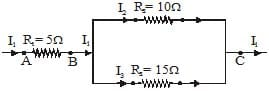(a) What is the potential difference across AB and across AC?

(b) What is the current through the other two resistors?

(c) What is the total resistance?

28. An electric bulb is rated at 200 V-100 W. What is its resistance? Five such bulbs burn for 4 hours. What is electrical energy consumed? Calculate the cost if the rate is 50 paise unit.  

29. State the formula co-relating the electric current flowing in a conductor and the voltage applied across it. Also show this relationship by drawing a graph.

What would be the resistance of a conductor if the current flowing through it is 0·35 ampere when the potential difference across it is 1·4 volt.  

30. (i) State the formula showing how the current I in a conductor varies when the potential difference V applied across it is increased stepwise  

(ii) Show this relationship also on a schematic graph.

(iii) Calculate the resistance of a conductor if the current flowing through it is 0·2 ampere when the applied potential difference is 0·8 volt.

31. When a potential difference of 1·2 volt is applied across a conductor, the current flowing through it is
0·25 ampere. Calculate the resistance of the conductor.  

32. A torch bulb is rated 5·0 V and 500 mA. Calculate its (i) power (ii) resistance and (iii) energy consumed when it is lighted for 4 hours.  

33. If a 12 V battery is connected to the arrangement of resistances given in Fig. (with 5 W replaced by 10 W, 15 W replaced by 5 W and 10 W replaced by 25 W). Calculate (i) the total effective resistance of the arrangement and (ii) the total current flowing in the circuit.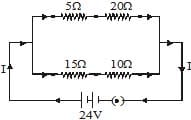34. Two electric lamps of 100 W and 25 W respectively are joined in parallel to a supply of 200 V. Calculate the total current flowing through the circuit. 

35. Two identical resistors, each of resistance 2 W, are connected in turn (i) in series, and (ii) in parallel, to a battery of 12 V. Calculate the ratio of power consumed in the two cases.  

36. Two identical resistors, each of resistance 10 W are connected in (i) series, and (ii) in parallel, in turn to a battery of 10 V. Calculate the ratio of power consumed in the combination of resistors in the two cases. 

37. In the given circuit, calculate (i) total resistance of the circuit, and (ii) current shown by the ammeter.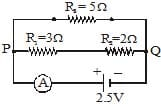38. (i) Draw a schematic diagram of a circuit consisting of a battery of five 2 V cells, a 5 W resistor, a 10 W resistor and a 15 W resistor, and a plug key, all connected in series. 

(ii) Calculate the current passing through the above circuit when key is closed.

39. In a household, 5 tube lights of 40 W each are used for 5 hours and an electric press of 500 W for 4 hour each day. Calculate the total energy consumed by the tube lights and press in a month of 30 days. 

40. In the circuit shown in Fig., calculate (a) total resistance in arm CE, (b) total current drawn from the battery, and (c) current in each arm, i.e., AB and CE of the circuit.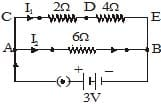41. (a) What is meant by 'Electric Resistance' of a conductor?  

(b) A wire of length L and resistance R is stretched so that its length is doubled and area of cross· section is halved. How will its : (i) resistance change? (ii) resistivity change?

42. (a) State Ohm's law.  

(b) Draw a schematic diagram of the circuit for studying Ohm's law.

43. Two lamps, one rated 60 W at 220 V and the other 40 W at 220 V, are connected in parallel to the electric supply at 220 V.  

(a) Draw a circuit diagram to show the connections.

(b) Calculate the current drawn from the electric supply.

(c) Calculate the total energy consumed by the two lamps together when they operate for one hour.

44. (a) Distinguish between the terms 'overloading' and 'short-circuiting' as used in domestic circuits. 

(b) Why are the coils of electric toasters made of an alloy than a pure metal ?

45. For the circuit shown in Fig., calculate  

(a) the value of current through each resistance

(b) the total current in the circuit

(c) the total effective resistance of the circuit.

Long Answer Questions (Carrying 5 Marks each)

46. (a) Express Ohm's law by a mathematical formula.  

(b) Draw a circuit diagram to verify Ohm's law.

(c) Present the relationship between the voltage applied across a conductor and the current flowing through it graphically.

47. State Ohm's law. Express it mathematically. Define SI unit of resistance. Derive an expression for the equivalent resistance of three resistors R1, R2 and R3 connected in series (or in parallel).  

48. (a) Express Ohm's law both by a mathematical formula and by a graph line.  

(b) State SI units of (i) resistance and (ii) resistivity.

(c) What will be the equivalent resistance of two resistors R1 and R2 (i) connected in series and (ii) connected in parallel.

49. (a) What is meant by saying that the potential difference between two points is 1 volt? Name a device that helps to measure the potential difference across a conductor.  

(b) Why does the connecting cord of an electric heater not glow hot while the heating element does ?

(c) Electrical resistivities of some substances at 20°C are given below:

Silver 1·60 × 10_8 W m

Copper 1·62 × 10_8 W m

Tungsten 5·20 × 10_8 W m

Iron 10·0 × 10_8 W m

Mercury 94·0 × 10_8 W m

Nichrome 100 × 10_6 W m

Answer the following questions in relation to them :

(i) Among silver and copper, which one is a better conductor? Why?

(ii) Which material would you advise to be used in electrical heating devices? Why?

50. (a) Name an instrument that measures electric current in a circuit. Define the unit of electric current. 

(b) What do the following symbols mean in circuit diagram?

(i)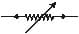(ii)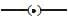(c) An electric circuit consisting of a 0·5 m long nichrome wire XY, an ammeter, a voltmeter, four cells of 1·5 V each and a plug key was set up.

(i) Draw a diagram of this electric circuit to study the

relation between the potential difference maintained between the points 'X' and 'Y' and the electric current flowing through XY.

(ii) Graph shown in Fig. was plotted between V and I values.

What would be the values of V/I ratios when the potential difference is 0·8 V, 1·2 V and 1·6 V respectively? What conclusion do you draw from these values?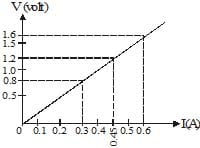The document Practice Questions: Electricity Notes | Study Science Class 10 - Class 10 is a part of the Class 10 Course Science Class 10.
All you need of Class 10 at this link: Class 10Use Code STAYHOME200 and get INR 200 additional OFF

## Science Class 10

107 videos|274 docs|162 tests

Track your progress, build streaks, highlight & save important lessons and more!

,

,

,

,

,

,

,

,

,

,

,

,

,

,

,

,

,

,

,

,

,

;